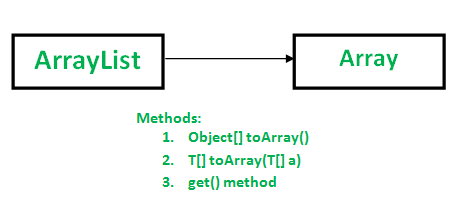# ArrayList to Array Conversion in Java : toArray() Methods

• Difficulty Level : Easy
• Last Updated : 07 Jul, 2022

Following methods can be used for converting ArrayList to Array:Method 1: Using Object[] toArray() method

Syntax:

``` public Object[] toArray()
```

• It is specified by toArray in interface Collection and interface List
• It overrides toArray in class AbstractCollection
• It returns an array containing all of the elements in this list in the correct order.

## Java

 `// Java program to demonstrate working of``// Objectp[] toArray()``import` `java.io.*;``import` `java.util.List;``import` `java.util.ArrayList;`` ` `class` `GFG {``    ``public` `static` `void` `main(String[] args)``    ``{``        ``List al = ``new` `ArrayList();``        ``al.add(``10``);``        ``al.add(``20``);``        ``al.add(``30``);``        ``al.add(``40``);`` ` `        ``Object[] objects = al.toArray();`` ` `        ``// Printing array of objects``        ``for` `(Object obj : objects)``            ``System.out.print(obj + ``" "``);``    ``}``}`

Output

`10 20 30 40 `

Note: toArray() method returns an array of type Object(Object[]). We need to typecast it to Integer before using as Integer objects. If we do not typecast, we get compilation error. Consider the following example:

## Java

 `// A Java program to demonstrate that assigning Objects[]``// to Integer[] causes error.``import` `java.io.*;``import` `java.util.List;``import` `java.util.ArrayList;`` ` `class` `GFG {``    ``public` `static` `void` `main(String[] args)``    ``{``        ``List al = ``new` `ArrayList();``        ``al.add(``10``);``        ``al.add(``20``);``        ``al.add(``30``);``        ``al.add(``40``);`` ` `        ``// Error: incompatible types: Object[]``        ``// cannot be converted to Integer[]``        ``Integer[] objects = al.toArray();`` ` `        ``for` `(Integer obj : objects)``            ``System.out.println(obj);``    ``}``}`

Output:

```19: error: incompatible types: Object[] cannot be converted to Integer[]
Integer[] objects = al.toArray();
^
1 error
```

It is therefore recommended to create an array into which elements of List need to be stored and pass it as an argument in toArray() method to store elements if it is big enough. Otherwise a new array of the same type is allocated for this purpose.

Method 2: Using T[] toArray(T[] a)

```// Converts a list into an array arr[] and returns same.
// If arr[] is not big enough, then a new array of same
// type is allocated for this purpose.
// T represents generic.
public  T[] toArray(T[] arr)
```

Note that the there is an array parameter and array return value. The main purpose of passed array is to tell the type of array. The returned array is of same type as passed array.

• If the passed array has enough space, then elements are stored in this array itself.
• If the passed array doesn’t have enough space, a new array is created with same type and size of given list.
• If the passed array has more space, the array is first filled with list elements, then null values are filled.

It throws ArrayStoreException if the runtime type of a is not a supertype of the runtime type of every element in this list.

## Java

 `// A Java program to convert an ArrayList to arr[]``import` `java.io.*;``import` `java.util.List;``import` `java.util.ArrayList;`` ` `class` `GFG {``    ``public` `static` `void` `main(String[] args)``    ``{``        ``List al = ``new` `ArrayList();``        ``al.add(``10``);``        ``al.add(``20``);``        ``al.add(``30``);``        ``al.add(``40``);`` ` `        ``Integer[] arr = ``new` `Integer[al.size()];``        ``arr = al.toArray(arr);`` ` `        ``for` `(Integer x : arr)``            ``System.out.print(x + ``" "``);``    ``}``}`

Output

`10 20 30 40 `

Note : If the specified array is null then it will throw NullpointerException. See this for example.

Method 3: Manual method to convert ArrayList using get() method

We can use this method if we don’t want to use java in built toArray() method. This is a manual method of copying all the ArrayList elements to the String Array[].

```// Returns the element at the specified index in the list.
public E get(int index)
```## Java

 `// Java program to convert a ArrayList to an array``// using get() in a loop.``import` `java.io.*;``import` `java.util.List;``import` `java.util.ArrayList;`` ` `class` `GFG {``    ``public` `static` `void` `main(String[] args)``    ``{``        ``List al = ``new` `ArrayList();``        ``al.add(``10``);``        ``al.add(``20``);``        ``al.add(``30``);``        ``al.add(``40``);`` ` `        ``Integer[] arr = ``new` `Integer[al.size()];`` ` `        ``// ArrayList to Array Conversion``        ``for` `(``int` `i = ``0``; i < al.size(); i++)``            ``arr[i] = al.get(i);`` ` `        ``for` `(Integer x : arr)``            ``System.out.print(x + ``" "``);``    ``}``}`

Output

`10 20 30 40 `

Method 4: Using streams API of collections in java 8 to convert to array of primitive int type

We can use this streams() method of list and mapToInt() to convert ArrayList<Integer> to array of primitive data type int

```int[] arr = list.stream().mapToInt(i -> i).toArray();
```

## Java

 `// Java program to convert a ArrayList to an array`` ` `// using streams()`` ` `import` `java.io.*;``import` `java.util.List;``import` `java.util.ArrayList;`` ` `class` `GFG {``    ``public` `static` `void` `main(String[] args)``    ``{``        ``List al = ``new` `ArrayList();``        ``al.add(``10``);``        ``al.add(``20``);``        ``al.add(``30``);``        ``al.add(``40``);`` ` `        ``// ArrayList to Array Conversion``        ``int``[] arr = al.stream().mapToInt(i -> i).toArray();`` ` `        ``for` `(``int` `x : arr)``            ``System.out.print(x + ``" "``);``    ``}``}`

Output

`10 20 30 40 `

Reference:
http://docs.oracle.com/javase/1.5.0/docs/api/java/util/ArrayList.html#toArray()
This article is contributed by Nitsdheerendra. If you like GeeksforGeeks and would like to contribute, you can also write an article using write.geeksforgeeks.org or mail your article to review-team@geeksforgeeks.org. See your article appearing on the GeeksforGeeks main page and help other Geeks.